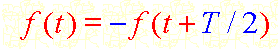# Fourier transform

magnifik
This isn't really a homework problem, but I am having trouble understanding why this is true:

A function with the following symmetry does not have any even harmonics in its spectrum.I understand the concept based on odd/even symmetry properties, but can anyone provide a mathematical proof?

## Answers and Replies

Staff Emeritus
$$a_{2n} = \frac{1}{T}\int_{-T/2}^{T/2} f(t) \cos 2n\omega t\,dt = \frac{1}{T}\left(\int_{-T/2}^{0} f(t) \cos 2n\omega t\,dt + \int_{0}^{T/2} f(t) \cos 2n\omega t\,dt\right)$$